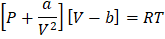# The pressure exerted by 1 mol ofat 273 K is 34.98 atm. Assuming that volume occupied bymolecules is negligible, the value of van der Waals’ constant for attraction ofgas is a)b)c)d)## Question ID - 102610 :- The pressure exerted by 1 mol ofat 273 K is 34.98 atm. Assuming that volume occupied bymolecules is negligible, the value of van der Waals’ constant for attraction ofgas is a)b)c)d)3537

 (a)OrSince,is constant at givenandcan have only one value or discriminant = 0